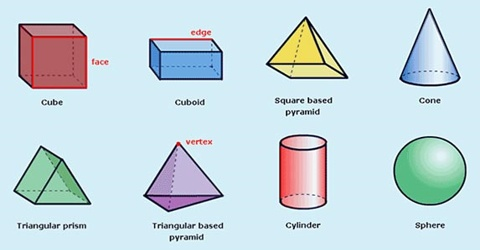Mathematic

# Solid Figures in GeometrySolid figures

Solid figures are three-dimensional figures that have length, width and height. Solid figures have a width, a depth, and a height. For example, look at your computer, laptop, phone, or whatever else you are using to view this lesson.

See some examples of three-dimensional figures below.Prism: Prism is a solid three-dimensional shape with two identical, parallel polygon bases. A prism is a figure made of two parallel faces that are polygons of the same shape and sides that are parallelograms.

Triangular prism: A prism with triangular faces with three-sided prism; it is a polyhedron made of a triangular base, a translated copy, and 3 faces joining corresponding sides.

Cube: A cube is a prism with squares for sides and faces. A cube has 6 equal and plane surfaces. All the faces of a cube are square in shape. In a cube there are 6 plane surfaces. There are 8 vertices and 12 edges.

Pyramid: A figure with a base that is a polygon and triangular sides. It is a polyhedron that has a base and three or more triangular faces that meet at a point

Cone: A cone has a circular base connected to a vertex. A cone has one plane circular surface, i.e. its base and only one curved surface.

Cylinder:  A cylinder stands on a circular plane surface having circular plane surfaces on its top and bottom. Cylinder has two equal circular bases that are parallel. It has a curved surface in the middle.

Sphere: A figure with a curved surface in which all points on the surface are equal distance from the center. In sphere there is curve surface, no edge and no vertex.

Octahedron: Octahedron is a three-dimensional shape having eight plane faces, especially a regular solid figure with eight equal triangular faces.